## UGC NET CS 2018-DEC Paper-2

 Question 1
​Data warehouse contains___________ data that is never found in operational environment.
 A Scripted B Encoded C Encrypted D Summary
Database-Management-System       Data-ware-housing
Question 1 Explanation:
Scripted data : A data that you access using java script is termed as scripted data.
Summary Data : Summary data means a new record is created to store values in a row of a relation.
 Question 2
Suppose a system has 12 instances of some resources with n processes competing for that resource. Each process may require 4 instances of the resource. The maximum value of n for which the system never enters into deadlock is
 A 3 B 4 C 5 D 6
Question 2 Explanation:
→ Here, every process requirement is 4 instances of the resource.
→ If we allocates 3 instance( one instance less than the requirement of each process) of the resource and to one process we allocate its minimum requirement then in that way with limited available instance of resource, without entering into deadlock we can fulfill requirement of maximum number of processes.
→ Now in question it is given that we have 12 instance then using above strategy we can allocate resources to 3 process without entering into deadlock.
 Question 3
​To overcome difficulties in Readers-Writers problem, which of the following statement/s is/are true?
Choose the correct answer from the code given below:
 A 1 only B Both 2 and 3 C 2 only D 3 only
Operating-Systems       Memory-Management
Question 3 Explanation:
 Question 4
Consider the following terminology and match List 1 and List 2 and choose the correct answer from the code given below
b= branch factor
d= depth of shallowest solution
M= Maximum depth of the search tree
I= depth limitA (a)-(iii), (b)-(ii), (c)-(iv), (d)-(i) B (a)-(ii), (b)-(iii), (c)-(iv), (d)-(i) C (a)-(i), (b)-(ii), (c)-(iv), (d)-(iii) D (a)-(i), (b)-(iii), (c)-(iv), (d)-(ii)
Algorithms       Time-Complexity
Question 4 Explanation:
BFS → O( b d ) worst case space complexity
DFS → O(bm) worst case space complexity
Depth- Limited Search → O(bl)
Iterative deepening Search → O(bd)
Note: Based upon BFS and DFS we can find the solution.
 Question 5
​The third generation mobile phone are digital and based on
 A AMPS B Broadband CDMA C CDMA D D-AMPS
Computer-Networks       CDMA
Question 5 Explanation:
→ AMPS, D-AMPS, CDMA, Broadband CDMA are the standard methods used for cellular communication.
→ ​ AMPS(Advanced Mobile Phone Service):​ It uses frequency division multiple access(FDMA) to provide access to the channel by multiple stations at the same time. In FDMA whole available bandwidth is divided into small frequency bands and each station is allocated to one frequency band which it will use to send and receive data.
→ ​ D-AMPS(Digital- Advanced Mobile Phone Service) :​ It uses time division multiple access(TDMA). In this kind of medium access all stations uses the whole available bandwidth for a fixed time slots.
→ ​ CDMA(Code Division Multiple Access) :​ In CDMA all stations are allowed to use the channel simultaneously and can use the the whole bandwidth. In this technique the sender and receiver uses a code to distinguish there data from others.
→ ​ Broadband CDMA :​ Broadband code division multiple access (B-CDMA) is a CDMA-based cell phone technology that uses broadband transmission. It is an improvement over the first-generation CDMA, which uses narrowband transmission. This kind of technology allows for better deployment of transmitter signals.
 Question 6
Consider ISO-OSI network architecture reference model. Session layer of this model offer Dialog control, token management and ____________ as services.
 A Synchronization B Asynchronization C Errors D Flow control
Computer-Networks       OSI-TCP-layers
Question 6 Explanation:
→ The session layer is the network dialog controller. It establishes, maintains, and synchronizes the interaction among communicating systems.
→ ​ Dialog control:​ The session layer allows two systems to enter into a dialog. It allows the communication between two processes to take place in either half-duplex (one way at a time) or full-duplex (two ways at a time) mode.
→ ​ Synchronization​ : The session layer allows a process to add checkpoints, or synchronization points, to a stream of data. For example, if a system is sending a file of 2000 pages, it is advisable to insert checkpoints after every 100 pages to ensure that each 100-page unit is received and acknowledged independently. In this case, if a crash happens during the transmission of page 523, the only pages that need to be reset after system recovery are pages 501 to 523. Pages previous to 501 need not be resent.
 Question 7
Consider the following two languages:
L​ 1​ = {x | for some y with | y| = 2 |x| ,xy∈ L and L is regular language}
L​ 2​ = { x | for some y such that |x| = |y|, xy∈ L and L is regular language}
Which one of the following is correct?
 A Both L​ 1​ and L​ 2​ are regular languages B Both L​ 1​ and L​ 2​ are not regular languages C Only L​ 1​ is regular language D Only L​ 2​ is regular language
Theory-of-Computation       Regular-Language
Question 7 Explanation:
if L is a regular language then we always have a string “w” which can be broken into “xy” such that |y|=2|x|
Consider a language L= {a​ 3n​ | n ≥ 0} , the strings in L are {ε, aaa, aaaaaa, .......}
then L​ 1​ = {a​ n​ | n ≥ 0} as every string in L can be broken into three equal parts .
DFA for LDFA for L​ 1By same logic L​ 2​ is also regular, here we have to break each string of L into two equal parts and also every even length string from L will belongs to L​ 1​ , since we can break only even length string into two equal parts such that |x| = |y| if “xy ε L”
 Question 8
​An internet service provider (ISP) has following chunk of CIDR-based IP addresses available with it: 245.248.128.0/20 . The ISP want to give half of this chunk of addresses to organization A and a quarter to Organization B while retaining the remaining with itself. Which of the following is a valid allocation of addresses to A and B?
 A 245.248.132.0/22 and 245.248.132.0/21 B 245.248.136.0/21 and 245.248.128.0/22 C 245.248.136.0/24 and 245.248.132.0/21 D 245.248.128.0/21 and 245.248.128.0/22
Question 8 Explanation:Question 9
​The Software Requirement Specification(SRS) is said to be _________ if and only if no subset of individual requirements described in it conflict with each other.
 A Correct B Consistent C Unambiguous D Verifiable
Software-Engineering       SRS
Question 9 Explanation:
The Software Requirement Specification(SRS) is said to be Consistent if and only if no subset of individual requirements described in it conflict with each other.
Unambiguous : ​ SRS said to be unambiguous if there exist no word that have more than one meaning and this will not confuse testers to get the exact reference.
Correct : SRS is said to be correct if it is error free and accurate.
 Question 10
​Consider the vocabulary with only four propositions A,B,C and D. How many models are there for the following sentence?
( ​ ⌐ ​ A ∨​ ⌐ ​ B ∨​ ⌐ ​ C ∨​ ⌐ ​ D)
 A 8 B 7 C 15 D 16
Engineering-Mathematics       Propositional-Logic
Question 10 Explanation:
Here, number of models is nothing but number of TRUEs in final statement. In this proposition logic we got total 15 number of models.Question 11
​ Which of the following statements is/are ​ false?
P: The clean-room strategy to software engineering is based on the incremental software process model.
Q: The clean-room strategy to software engineering is one of the ways to overcome “unconscious” copying of copyrighted code.
Choose the correct answer from the code given below:
 A Neither P and Q B P only C Both P and Q D Q only
Software-Engineering       Software-process-models
Question 11 Explanation:
Clean-room Strategy:
Cleanroom software engineering (CSE) is a process model that removes defects before they can precipitate serious hazards. It is a team-oriented, theory based software, which is developed using the formal methods, correctness verification and Statistical Quality Assurance (SQA).
→ Clean room management is based on the incremental model of software development, which accumulates into the final product. The approach combines mathematical-based methods of software specification, design and correctness verification with statistical, usage-based testing to certify software fitness for use.
→ The main goal of clean room engineering is to produce zero error-based software by allowing correct designs, which avoid rework.
 Question 12
​If the frame buffer has 10-bits per pixel and 8-bits are allocated for each of the R,G and B components then what would be the size of the color lookup table(LUT)
 A (2​ 10​ +2​ 11​ ) bytes B (2​ 10​ +2​ 8​ ) bytes C (2​ 10​ + 2​ 24​ ) bytes D (2​ 8​ + 2​ 9​ ) bytes
Computer-Graphics       LUT
Question 12 Explanation:
10-bits per pixel it means we will have 2​ 10 ​ entries in color lookup table.
8-bits are allocated for each of the R,G and B components, means each entry in color lookup table is of 24-bits( 8-bits for each of the R, G and B component).
24-bits = 3 Bytes
So the size of lookup table is = Number of entries * size of each entry
So the size of lookup table is = (2​ 10​ * 3) Bytes
the size of lookup table is = 3072 Bytes = (2​ 10​ + 2​ 11​ ) Bytes
 Question 13
Suppose P,Q and R are co-operating processes satisfying Mutual Exclusion condition. Then if the process Q is executing in its critical section then
 A ‘P’ executes in critical section B ‘R’ executes in critical section C Neither ‘P’ nor ‘Q’ executes in their critical section D Both ‘P’ and ‘R’ executes in critical section
Question 13 Explanation:
● A mutual exclusion (mutex) is a program object that prevents simultaneous access to a shared resource.
● This concept is used in concurrent programming with a critical section, a piece of code in which processes or threads access a shared resource.
● Only one thread owns the mutex at a time, thus a mutex with a unique name is created when a program starts.
● When a thread holds a resource, it has to lock the mutex from other threads to prevent concurrent access of the resource
● In the question, Three process are cooperating processes and satisfying mutual exclusion condition. If process Q is executing in its critical section means remaining two processes in wait stating and they won’t enter into critical section.
 Question 14
A Computer uses a memory unit with 256K word of 32 bits each. A binary instruction code is stored in one word of memory. The instruction has four parts: an indirect bit, an operation code and a register code part to specify one of 64 registers and an address part. How many bits are there in operation code, the register code part and the address part?
 A 7,7,18 B 18,7,7 C 7,6,18 D 6,7,18
Operating-Systems       Memory-Management
Question 14 Explanation:
An instruction size is given as 32-bits.
Now, the instruction is divided into four parts :
An indirect bit
Register code part : Since number of registers given as 64(2​ 6​ ) so to identify each register uniquely 6-bits are needed.
Address part : 256K(2​ 18​ ) word memory is mentioned so to identify each word uniquely 18-bits are needed.
Operation code:
Size of Operation code = Complete instruction size - (size of indirect bit + size of register code + size of address part)
Size of Operation code= 7-bits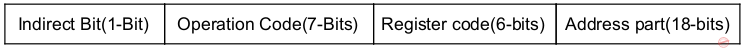Question 15
​Consider the following set of processes and the length of CPU burst time given in milliseconds:Assume that processes being scheduled with Round-Robin Scheduling Algorithm with Time Quantum 4ms. Then The waiting time for P4 is _________ ms.
 A 0 B 4 C 12 D 6
Operating-Systems       CPU-Scheduling
Question 15 Explanation:
Waiting time = Turnaround time- Burst time
Turnaround time = Completion time - Arrival time.
Since in question Arrival time is not given so consider it as “0” for all the processes .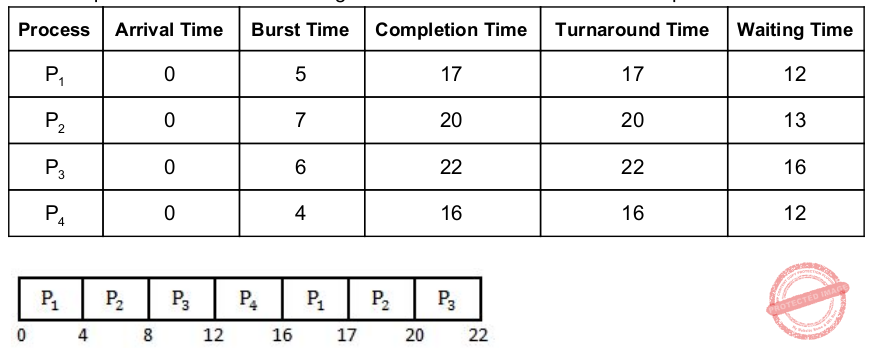Question 16
Which of the following HTML5 codes will affect the horizontal as well as vertical alignment of the table content?
 A < t d halign = " middle" valign = " center" > BASH < / td > B < t d style = " text − a lign : center; vertical − a lign : middle; " > BASH < / td > C < t d align = "middle" valign = " center" > B ASH < / td > D < t d style = " horizontal − a lign : c enter; vertical − a lign : m iddle; " > B ASH < / td >
Web-Technologies       HTML
Question 16 Explanation:
● The text-align property sets the horizontal alignment (like left, right, or center) of the content in or .
● The vertical-align property sets the vertical alignment (like top, bottom, or middle) of the content in or .
 Question 17
The number of substrings that can be formed from string given by “a d e f b g h n m p” is
 A 10 B 45 C 56 D 55
Theory-of-Computation       Languages-and-Grammars
Question 17 Explanation:
If we have no repetition in a string then the number of substrings can be found using the formula :
n*(n+1)/2 + 1
We have added 1 because it may include a NULL string also.
The number of substrings = 10*(11)/2 +1
The number of substrings = 56
 Question 18
​Which of the following statement/s is/are true?
(i) Firewall can screen traffic going into or out of an organization.
(ii) Virtual private networks cam simulate an old leased network to provide certain desirable properties.
Choose the correct answer from the code given below:
 A (i) only B Neither (i) nor(ii) C Both (i) and (ii) D (ii) only
Computer-Networks       Firewall
Question 18 Explanation:
Statement 1 is correct because firewall works on the Application layer, so it can screen the traffic going into and out of the traffic.
Statement 2 is correct.Virtual private networks cam simulate an old leased network to provide certain desirable properties.
 Question 19
Match the List 1 and List 2 and choose the correct answer from the code given belowA (a)-(i), (b)-(ii), (c)-(iii), (d)-(iv) B (a)-(ii), (b)-(i),(c)-(iii), (d)-(iv) C (a)-(iv), (b)-(iii), (c)-(ii), (d)-(i) D (a)-(iii), (b)-(iv), (c)-(ii), (d)-(i)
Engineering-Mathematics       Prepositional-Logic
Question 19 Explanation:
Propositions r and s are logically equivalent if the statement r ↔ s is a tautology. ∼q⇒ ∼p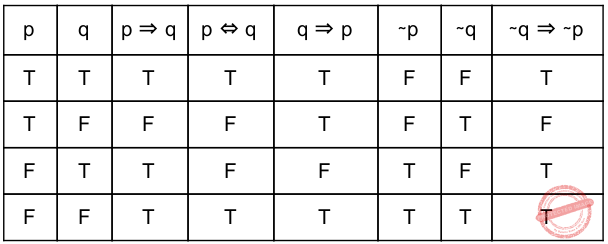According to above table,
equivalence means p ⇔ q
Contrapositive means p⇒ q : ∼q⇒ ∼p
Converse means p⇒ q : q⇒ p
Implication means p ⇔ q
 Question 20
Consider the following language:
L​ 1​ = { a n+m b n a m | n, m ≥ 0 }
L​ 2​ = { a n+m b n+m a n+m |n, m ≥ 0 }
Which one of the following is correct?
 A Only L​ 1​ is Context Free Language B Both L​ 1​ and L​ 2​ are not Context Free Language C Only L​ 1​ is Context Free Language D Both L​ 1​ and L​ 2​ are Context Free Language
Theory-of-Computation       Context-Free-Language
Question 20 Explanation:
→ L​ 1​ is Context Free language because we can push all the a’s that come as input and when b’s come as input pop “n” number of a’s for “n” b’s from top of the stack and when again a’s come as input pop-up all the remaining a’s(i.e. “M” number of a’s) from top of the stack for “m” number of a’s coming as input.
Since we can have a pushdown automata for L​ 1​ so we can say L​ 1​ is a CFL.
→ L​ 2​ is not a context free language because L is equivalent to language= {a​ p​ b​ p​ a​ p​ | p ≥ 0 }.
So for every “b” we can POP a’s came before “b” but for “a” which came after “b” we have nothing to POP on the top of stack. Since we can’t have a pushdown automata that can accept L​ 2​ .
L​ 2​ is not a CFL.
 Question 21
Consider the following statements related to AND-OR Search algorithm.
S​ 1​ : A solution is a subtree that has a goal node at every leaf.
S​ 2​ : OR nodes are analogous to the branching in a deterministic environment
S​ 3​ : AND nodes are analogous to the branching in a non-deterministic environment.
Which one of the following is true referencing the above statements?
Choose the correct answer from the code given below:
 A S1- False, S2- True, S3- True B S1- True, S2- True, S3- True C S1- False, S2- True, S3- False D S1- True, S2- True, S3- False
Engineering-Mathematics       Graph-Theory
Question 21 Explanation:
● An and–or tree is a graphical representation of the reduction of problems (or goals) to conjunctions and disjunctions of subproblems (or subgoals).
● A solution in an AND-OR tree is a sub tree whose leaves are included in the goal set
 Question 22
Which homogeneous 2D matrix transforms the figure (a) on the left side to the figure (b) on the right ?AB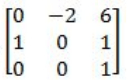CD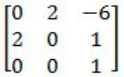Computer-Graphics       2-D-Geometrical-Transforms-and-Viewing
Question 22 Explanation:
The homogeneous coordinates of the cartesian point (x,y) is (x,y,1) and the transformation Matrix is a 3x3 Matrix.
The image in figure ​ a ​ is translated(by 1 unit in x axis), scaled( 2 units in y axis) and rotated(90 degrees counterclockwise) to figure in b.
 Question 23
​Identify the correct sequence in which the following packets are transmitted on the network by a host when a browser requests a web page from a remote server, assuming that the host has been restarted.
 A HTTP GET request, DNS query, TCP SYN B DNS query, TCP SYN, HTTP GET request C TCP SYN, DNS query, HTTP GET request D DNS query, HTTP Get request, TCP SYN
Computer-Networks       Transmission-and-Propagation-Delay
Question 23 Explanation:
Sequence in which the given packets are transmitted on the network by a host when a browser requests a web page from a remote server, assuming that the host has been restarted :
Step 1: DNS query is sent to Domain name server to convert the Domain name into it’s IP address.
Step 2: TCP SYN request packet is sent by sender to establish a connection for data transmission.
Step 3: HTTP GET request is used to request data from a specified IP address.
 Question 24
Consider a system with 2 level cache. Access times of Level 1, Level 2 cache and main memory are 0.5 ns, 5 ns and 100 ns respectively. The hit rates of Level1 and Level2 caches are 0.7 and 0.8 respectively. What is the average access time of the system ignoring the search time within cache?
 A 20.75 ns B 7.55 ns C 24.35 ns D 35.20 ns
Operating-Systems       Memory-Management
Question 24 Explanation:
Average access time = level 1 hit rate( level 1 access time)+ (level1 miss rate)(level 2 hit rate(level 2 access time)+ (level 1 miss rate)( level 2 miss rate) (main memory access time)
Average access time = 0.7(0.5)+ 0.3(0.8)(5)+ 0.3(0.2)(100)
Average access time = 7.55 ns
 Question 25
​ In computers, subtraction is generally carried out by
 A 1’s complement B 10’s complement C 2’s complement D 9’s complement
Digital-Logic-Design       Number-System
Question 25 Explanation:
● In computers, subtraction is generally carried out by 2’s complement.
● In two's-complement representation, positive numbers are simply represented as themselves, and negative numbers are represented by the two's complement of their absolute value;
● In the subtraction there may possibility of negative number as a result.
There are 25 questions to complete.

Register Now